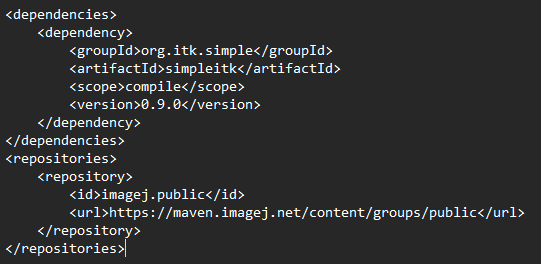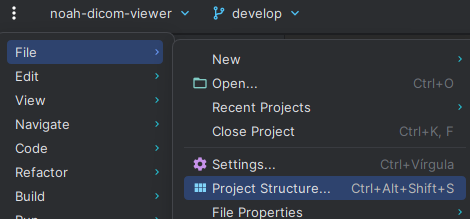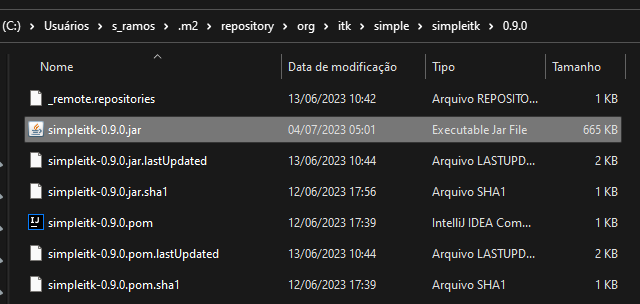# Java Convert int[] to VectorUInt32

The SImpleITK.extract method requires 3 parameters:

first is org.itk.simple.Image
second is org.itk.simple.VectorUInt32
third is org.itk.simple.VectorInt32


i know whats are this parameters, i was able to reproduce this in python.
But i dont know how to convert an int array to VectorUInt32 and VectorInt32, example:

int[] array = {512, 512, 0};
VectorUInt32 vectorUInt = new VectorUInt32(array, array, array);
VectorInt32 vectorInt = new VectorInt32(array, array, array);


VectorUInt32 and VectorInt32 has 3 constructors methods each.

VectorInt32(long l, boolean b) or
VectorInt32() or
VectorInt32(long l)


and

VectorUInt32(long l, boolean b) or
VectorUInt32() or
VectorUInt32(long l)


So how i’m supposed to convert this? It is very confusing.

Hello @Salu_Ramos,

I’m not too familiar with the Java version, but have you tried:

int[] array = {512,512,1}
VectorUInt32 vectorUInt = new VectorUInt32( array );
VectorInt32 vectorInt = new VectorInt32( array );


Note that the array size with third component of zero is usually not appropriate for image size. If in 2D size is 512\times512, if in 3D then 512\times512\times1.

if i try this, with array being a int[] or double[] or long[]:

VectorUInt32 vectorUInt = new VectorUInt32( array );

then:
java: incompatible types: int[] cannot be converted to long
java: incompatible types: double[] cannot be converted to long
java: incompatible types: long[] cannot be converted to long

tried this also:

public Image simpleSlice (long[] sliceSize, int[] sliceIndex) {
VectorUInt32 vectorSize = new VectorUInt32(); //like {512, 512, 0}
vectorSize.set(0, sliceSize);
vectorSize.set(1, sliceSize);
vectorSize.set(2, sliceSize);
VectorInt32 vectorIndex = new VectorInt32(); //like {0, 0, 10}
vectorIndex.set(0, sliceIndex);
vectorIndex.set(1, sliceIndex);
vectorIndex.set(2, sliceIndex);
Image sliceExtraction = SimpleITK.extract(this.volume, vectorSize, vectorIndex);
System.out.println(sliceExtraction);
return sliceExtraction;
}

then:
java.lang.UnsatisfiedLinkError: 'void org.itk.simple.SimpleITKJNI.VectorUInt32_set(long, org.itk.simple.VectorUInt32, int, long)'

Nothing works

i find this: Hello World — SimpleITK 2.0rc2 documentation

as in example:

long[] isize = {128, 128};
VectorUInt32 imageSize = new VectorUInt32( isize )

Documentation code does not work.

I just built and ran the HelloWorld example in Java on my PC, and it worked just fine. What version of Java are you using and on what platform? I’m using JDK 17.

1 Like

i’m running java 20.0.1 2023-04-18 in windows 11, with intellij idea community.

my pom.xml:i also added the binary folder in the “project structure” > “libraries”my VM options for binary path:

-Djava.library.path="D:\my github repos\noah-viewer-java\SimpleITK-2.3.0rc1.post2-Java-win64"

i downgraded jdk to 17 but got same error:

long[] isize = {128, 128};
VectorUInt32 imageSize = new VectorUInt32( isize );


java: incompatible types: long[] cannot be converted to long

Maybe my pom.xml is using an too old version? (0.9.0) I was unable to find another public maven repository that has newer version of simpleitk. If you can provide it to me @dchen i would be very grateful.

I’m not a real Java person, so I have no knowledge about Maven. Trying to read up on it. Looking at scijava maven repo, the simpleitk maven file seems to be just an XML file with a version number in it. I don’t see a jar file there, so how does that even work?

And, of course, the SimpleITK version there is very old.

I must say that I am not a java guy either, it just so happens that Java was the best alternative for the project I am working on now.

It turns out that the only maven repository that contained simpleITK only had it in a very old version, which I must say is far from being functional. I went to the folder where maven downloads the dependencies (.m2\repository\org\itk\simple\simpleitk\0.9.0), deleted the old jar file from version 0.9.0 and put the new one from version 2.3.0 with the name of version 0.9.0. This solved all the conversion problems. But of course this is a package management problem and after talking to a java expert friend of mine, I decided to start using gradle. And since there is no up-to-date simpleitk repository I will have to ask gradle to use a local folder as a dependency.picture of simpleitk-2.3.0 undercover as simpleitk-0.9.0

But here’s a new question @dchen, how did you configure your dependencies to run?

I use cmake to configure and build the SimpleITK source code. In the process it configures all the dependencies.

1 Like